In calculating percentages in Excel, there are a lot of ways that you could do this:

• What is the percentage of a number?
• What is the percentage change of value i.e. The percentage increase or decrease
• What is the proportion against a total value?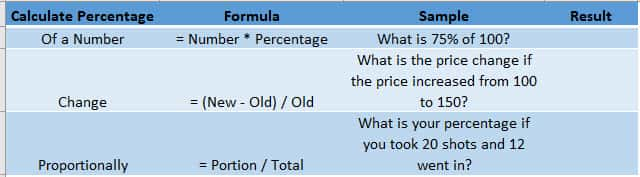Let us go over each method in the tutorial below:STEP 1: What is the percentage of a number?

To calculate the percentage of the number, simply multiply the number and the percentage together:

## 75% * 100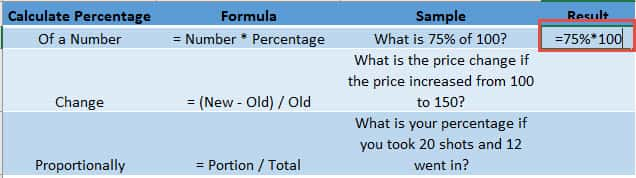STEP 2: What is the percentage change of value i.e. The percentage increase or decrease

To calculate the change in value as a percentage, get the difference of the new value and the old value, then divide it by the old value:

## (150 – 100 ) / 100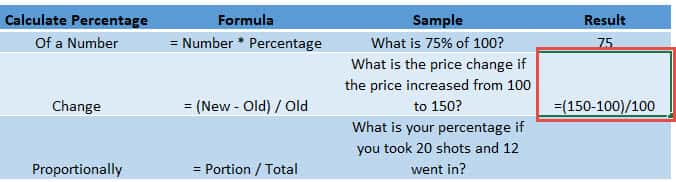STEP 3: What is the proportion against a total value?

To get the proportion as a percentage, divide the portion by the total amount:

## 12/20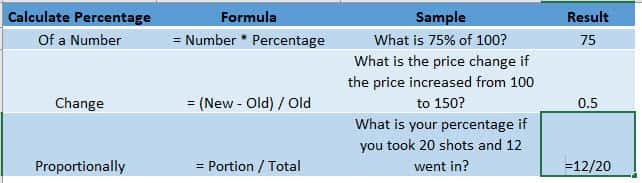STEP 4: We are almost done! The last two values are not in the percentage format, so let’s fix that.

Select the last two values and go to Home > Number > %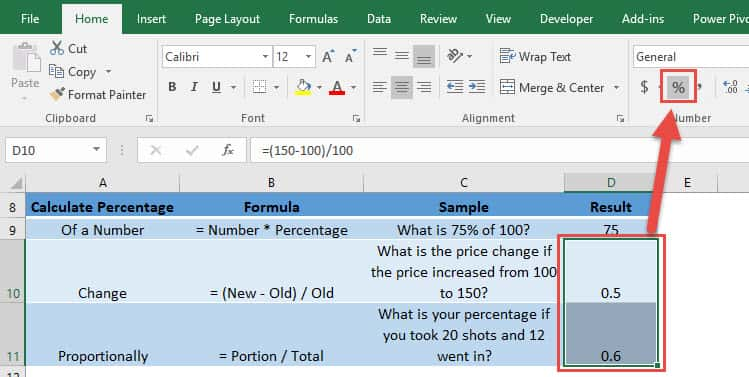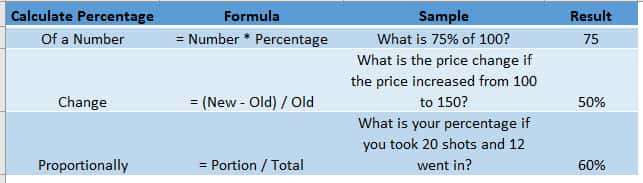How to Calculate Percentage in Excel